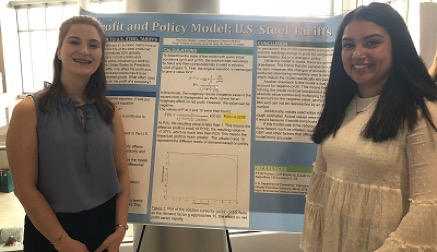# Mathematics

A degree in Mathematics from RWU is an excellent foundation for graduate study and a wealth of careers in fields such as science, computers, engineering and business. Our program blends a rigorous study of math theory with flexible electives and research conducted with faculty. You’ll learn how to interpret data and use mathematical reasoning to analyze and solve problems.

The Mathematics program at Roger Williams University provides preparation for graduate study and for a variety of careers in industry and government. There is enough flexibility in the program to allow a large choice of electives, and the program, when combined with further study in a second area, can provide an excellent foundation for graduate or professional study in the physical sciences, computer science, engineering or business. Our best students have opportunities for undergraduate research, while others write theses, present at national conferences and co-author journal articles with our faculty.

Several factors distinguish Roger Williams University from the competition. For starters, small class size means more personalized attention from our faculty members. Our Math major is made up of only 10 courses, leaving plenty of room for a double major, or to experience a wide variety of electives. Our students are involved on campus—as math tutors and as members of the Mathematics Honor Society. Unlike at other institutions, our talented students get to co-author math publications with our faculty. In fact, our students are currently working on the Numerical Solution of the Helmholtz Equation for the Pseudosphere, and Foundations of General Relativity and some speculations on the Einstein-Grossmann Collaboration. Recently, one of our students was awarded the top prize in the MAA Student Essay in History of Mathematics.

## Degree Requirements

### The Mathematics Major

Click to Open

Majors pursuing a Bachelor of Science in Mathematics must satisfy University Core Curriculum requirements and the College speech requirement, COMM 210. In addition, they must complete the following courses and a sufficient number of electives to total 120 credits. Majors are encouraged to apply electives toward a minor or second major.

SubjectNumberTitle
MATH213Calculus I and Lab
MATH214Calculus II and Lab
MATH221Discrete Mathematics
MATH331Linear Algebra
MATH351Calculus of Several Variables
MATH371Real Analysis
MATH390Abstract Algebra
MATH421Problem Seminar
and two electives selected from: MATH 255, 301, 305, 315, 317, 330, 335, 340, 342, 370, 381, 431

### The Mathematics Minor

Click to Open
SubjectNumberTitle
MATH213Calculus I and Lab
MATH214Calculus II and Lab
and 10 additional mathematics credits (200 level or above)

Engineering students who wish to pursue a math minor would benefit by taking three of the following courses:

SubjectNumberTitle
MATH255Introduction to Mathematical Software
MATH305Mathematical Modeling
MATH315Probably and Statistics
MATH317Differential Equations
MATH331Linear Algebra
MATH330Engineering Mathematics
or
MATH351Calculus of Several Variables
MATH342Numerical Analysis

Business students who wish to pursue a math minor would benefit by taking three of the following courses:

SubjectNumberTitle
MATH301Linear Programming
MATH315Probability and Statistics
MATH317Differential Equations
MATH331Linear Algebra
MATH342Numerical Analysis

Science students who wish to pursue a math minor would benefit by taking three of the following courses:

SubjectNumberTitle
MATH255Introduction to Mathematical Software
MATH305Mathematical Modeling
MATH315Probably and Statistics
MATH317Differential Equations
MATH331Linear Algebra
MATH342Numerical Analysis
MATH351Calculus of Several Variables

Computer Science students who wish to pursue a math minor would benefit by taking three of the following courses:

SubjectNumberTitle
MATH221Discrete Mathematics
MATH315Probably and Statistics
MATH331Linear Algebra
MATH342Numerical Analysis
MATH390Abstract Algebra

### The Mathematics and Secondary Education Dual Major

Click to Open

Majors pursuing a dual major for secondary education must satisfy University Core Curriculum requirements and the College speech requirement, COMM 210. In addition, they must complete the following courses and a sufficient number of electives to total 120 credits.

*Note: There are no electives among the mathematics courses required for the double major with secondary education.

SubjectNumberTitle
MATH213Calculus I and Lab
MATH214Calculus II and Lab
MATH221Discrete Mathematics
MATH315Probability and Statistics
MATH331Linear Algebra
MATH335Topics for Secondary Mathematics Education
MATH340History of Mathematics
MATH351Calculus of Several Variables
MATH390Abstract Algebra
COMSC110Introduction to Computer Science I and Lab

### Student Learning Outcomes

Mathematics majors at RWU are expected to have the ability to:

• Prove classical theorems at the appropriate level of rigor
• Reason mathematically
• Read mathematical texts and articles with understanding
• Write answers, proofs, and papers in appropriate mathematical style
• Use appropriate technology successfully
• Analyze problems and choose the correct technique from their repertoire to solve them
• Make inferences and generalizations

Mathematics /Secondary Education majors at RWU are expected to have the same abilities.Stephanie Brooks '20, a Business Management major and Lorena Cambizaca '19 a Biology major and Math minor presented their Differential Equations poster "Profit and Policy Model: U.S. Steel Tariffs" at the 2018 Student Academic Showcase and Honors event at RWU.

### Research Projects

• “The Libra Astronomica and Its Mathematics”
• “The Numerical Solution for the Helmholtz Equation for the Superellipsoid-Mars Project”
• “Fixed Points in Affine Cipher”
• “Does Microsoft Sales Help Drive the Client to Upgrade their Operating System (Windows)”
• “The Foundations of General Relativity & Some Speculations on Einstein-Grossman Collaboration”

### Employers

(partial list)

• AT&T
• School Districts (Various)
• Raytheon
• CA Simmons Associates
• Fram Corporation
• HBO Company
• Wall Street, NYC
• NY State Department of Health

(partial list)

• Brown University
• Dartmouth College
• Harvard University
• Georgia Institute of Technology
• University of California-Irvine
• University of Wisconsin
• Boston College
• SUNY - Albany
• New York University
• Cornell University
• Tufts University
• Texas A&M
##### RISE STEM Scholarship at RWURWU offers a scholarship opportunity for students interested in majoring in math, sciences, engineering, computer science or forensic science. Learn about the National Science Foundation RISE Scholars program, which recognizes, supports and empowers a group of academically talented students with financial need as they prepare to enter Research and Industry in Science and Engineering (RISE).